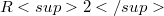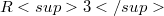﻿

### A class of topological space geometries

#### Abstract

The purpose of this paper is to introduce$R2$-divisible$R3$-spaces. This is a branch of topological space geometries in the sense of Betten. We define a few more topologies on the line set in$R2$-divisible$R3$-spaces and investigate under the consequences of these topologies basic properties of these space geometries.

DOI Code: 10.1285/i15900932v15n2p175

Keywords: Topological geometry; Space geometry

Classification: 51H10

Full Text: PDF